Mobile QR Code1. (Department of semiconductor science, Dongguk University, Seoul, 04620, Korea)
2. (Department of POLED circuit research team, LG Display, Seoul, 07796, Korea)

AMOLED displays, charge-transfer circuit, feedback trans-conductance current-mode driver, ultra-low compensation current

I. INTRODUCTION

Recently, active matrix organic light-emitting diode (AMOLED) displays have received great attention because of their high contrast ratio, fast response time, and low thickness compared to other displays. AMOLEDs, which are current-driven devices, require current-driving transistors such as thin film transistors (TFT) to flow stable and programmable current through OLEDs. However, TFTs are vulnerable to threshold-voltage and mobility variations because of device mismatches, which produce significant non-uniformity in displays (1). Two types of drivers exist to compensate current variations from such process variations: the voltage-driving method and current-driving method. The voltage-driving method is widely used for large displays because of its high driving speed. However, this approach needs additional in-pixel circuits to sample and compensate for threshold-voltage variations (${Δ}$V$_{\mathrm{TH}}$). Furthermore, the voltage-driving method can compensate for ${Δ}$V$_{\mathrm{TH}}$ but not mobility variations (${Δ}$${μ}) (2,3). On the other hand, the current-driving method, which consists of a trans-conductance (FB-Gm) circuit, has better luminance uniformity than the voltage-driving method because the former can compensate for {Δ}$${μ}$ and ${Δ}$V$_{\mathrm{TH}}$. However, this approach requires longer compensation times compared to the voltage-driving method, especially under low-luminance conditions, because of the significant parasitic elements of arrays.

In this paper, we propose a new low-luminance compensation current driver with a charge transfer circuit for mobile and automobile applications that require double-wide ultra XGA (DWUXGA) AMOLED displays to obtain the precise compensation of the current mismatch with high driving speed. With this proposed current driver, both ${Δ}$V$_{\mathrm{TH}}$ and ${Δ}$${μ}$ can be accurately compensated with high driving speed by using an initialization technique with a charge-transfer circuit.

Fig. 1. Conventional FB-Gm Circuit (a) Schematic, (b) and transient simulation result (V$_{\mathrm{DATA }}$: 1 V).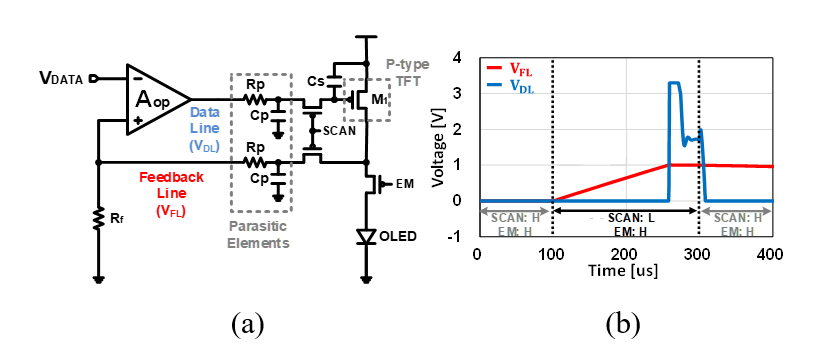Fig. 2. Loop stability of FB-Gm using an ideal OPA (a) Loop gain, (b) Loop phase.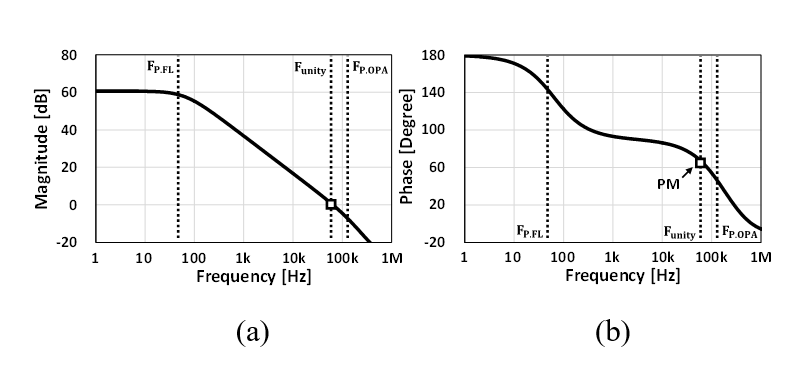II. Conventional fb-gm circuit

Fig. 1(a) shows a current driver that uses a conventional FB-Gm circuit that consists of an op-amp (OPA) fabricated from CMOS and a p-type TFT, an OLED, and switches fabricated from TFTs. Parasitic elements (R$_{\mathrm{P}}$, C$_{\mathrm{P}}$) from the pixel array must be considered because these features affect timing-related specifications such as the one-row time, indicating how quickly current compensation is completed.

The operation of conventional FB-Gm is as follows. During the compensation phase, SCAN switches are turned on while EM switches are turned off. When the data voltage (V$_{\mathrm{DATA}}$) increases, the data-line voltage (V$_{\mathrm{DL}}$) decreases because of the polarity of OPA. Thus, the current of the driving transistor (M$_{1}$) and the feedback-line voltage (V$_{\mathrm{FL}}$) increase, up to being equivalent to V$_{\mathrm{DATA}}$. The current of M$_{1}$ is determined by the feedback resistor (R$_{\mathrm{f}}$), and the gate voltage of M$_{1}$ (V$_{\mathrm{G,M1}}$) is sampled by the storage capacitor (C$_{\mathrm{S}}$) during the compensation phase. During the emission phase, SCAN switches are turned off, EM switches are turned on, and M$_{1 }$can flow the programmed current in the OLED with the sampled voltage. R$_{\mathrm{f}}$ represents OLEDs and is inversely proportional to the current, so we used 100 M${Ω}$ for R$_{\mathrm{f}}$ when V$_{\mathrm{DATA}}$ was 1 V and the current was 10 nA. As shown in Fig. 1(b), the low initial voltage of the feedback line in the emission phase, which was close to 0 V, necessitated 150 ${\mathrm{\mu}}$s of extra time to pull up 1 V of V$_{\mathrm{FL}}$. To lower the one-row time below 10 ${\mathrm{\mu}}$s for AMOLED displays with 60 Hz of frequency and 1200 vertical lines, the pull-up time should be reduced. In terms of the settling time, loop stability should also be considered because conventional FB-Gm with p-type TFT is a cascaded structure that consists of an OPA and p-type common-source amplifier (CSA). The key ac parameters of FB-Gm are as follows:

(1)
$A_{L}=g_{m . M 1} \cdot A_{O P} \cdot R_{f}$

(2)
$F_{P . F L}=\frac{1}{2 \pi \cdot\left[r_{o, M 1} \cdot\left(1?+ g_{m . M 1} \cdot A_{o p} \cdot R_{f}\right) / / R_{f}\right] \cdot C_{p}} \approx \frac{1}{2 \pi \cdot R_{f} \cdot C_{p}}$

(3)
$F_{\text {unity }}=A_{L} \cdot F_{P . F L},$ where $F_{P . O P A} \square F_{\text {unity}}$

where A$_{\mathrm{L}}$ is the loop gain, g$_{\mathrm{m.M1}}$ is the trans-conductance of M$_{1}$, A$_{\mathrm{OP}}$ is the open-loop gain of the OPA, F$_{\mathrm{P, FL}}$ is the pole of the feedback line, r$_{\mathrm{o.M1}}$ is the output impedance of M$_{1}$, and C$_{\mathrm{p}}$ is the parasitic capacitance in the feedback line. As given by (2), the dominant pole is F$_{\mathrm{P, FL}}$ because of the high output impedance at the feedback line and the parasitic capacitance.

Fig. 2 shows the loop stability for FB-Gm. For stable operation with a good phase margin (PM), the internal pole of the OPA (F$_{\mathrm{P, OPA}}$) should appear at a higher frequency than the unity-gain frequency (F$_{\mathrm{unity}}$). Additionally, the FB-Gm should have a high F$_{\mathrm{unity}}$, which is the function of A$_{\mathrm{OP}}$ in (3), to achieve fast settling time, which should produce an OPA with high performance.

To solve the issues of conventional FB-Gm circuits regarding implementation in current drivers, such as long pull-up times and the difficulty of creating high-performance OPAs, we propose an initialization technique with a charge-transfer circuit in an FB-Gm current-mode driver structure.

III. Circuit Description

Fig. 3 shows the proposed current driver based on FB-Gm with an initialization technique. In the panel, a 4T1C pixel circuit is embodied in each panel pixel for external compensation. The silicide resistor, R$_{\mathrm{f}}$, is limited by the range of the programmed current and needs a huge layout area for fabrication. Therefore, we changed R$_{\mathrm{f}}$ into a diode-connected nMOS (M$_{2}$) with a source-degeneration resistor (R$_{\mathrm{deg}}$) for its wide range of programmed currents, small area, and good linearity. Three different compensation phases exist for operation as a current driver: the first initialization, the second initialization, and the compensation phases. During the first initialization phase, the capacitor (C$_{1}$) samples V$_{\mathrm{DATA}}$ and the other capacitor (C$_{2}$) has no charge because the OPA operates as an analog buffer, as shown in Fig. 4(a). Therefore, the feedback line and data line are initialized as V$_{\mathrm{DATA}}$. In the second initialization phase, all the charges in C$_{1}$ transfer to C$_{2}$, and the function of V$_{\mathrm{DL}}$ is as follows:

Fig. 3. Proposed FB-Gm structure with an initialization function.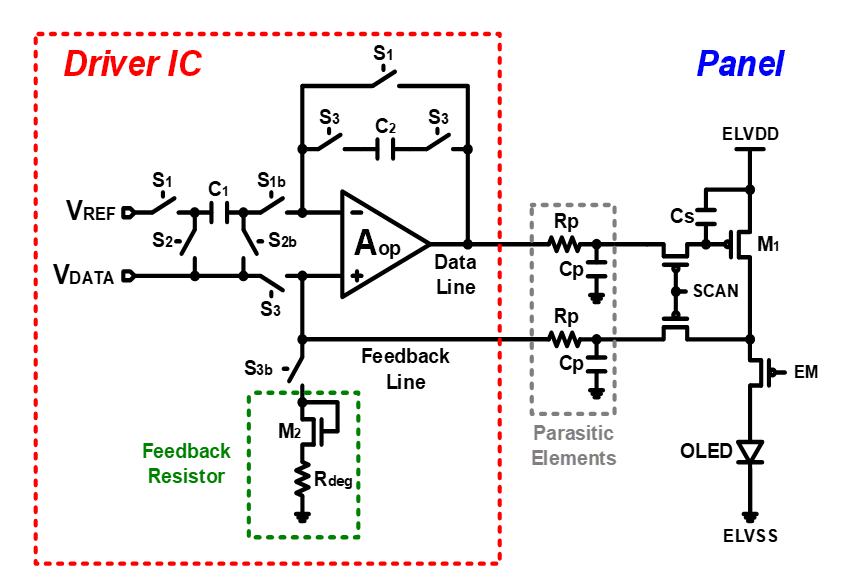Fig. 4. Proposed initialization function (a) First initialization phase for the feedback line (S1: on, S2: off), (b) Second initialization phase for the data line (S1: off, S2: on).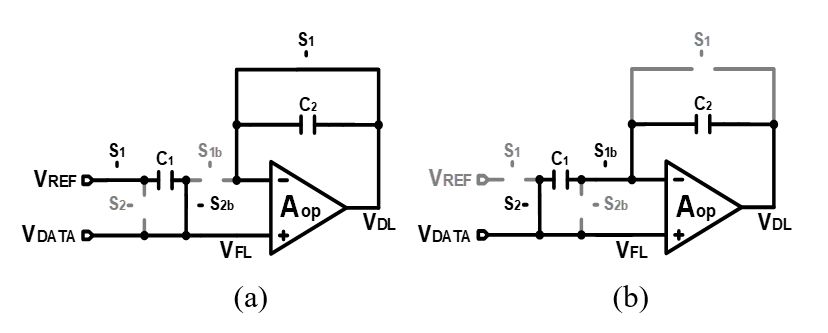Fig. 5. Simulation result of the proposed initialization circuit.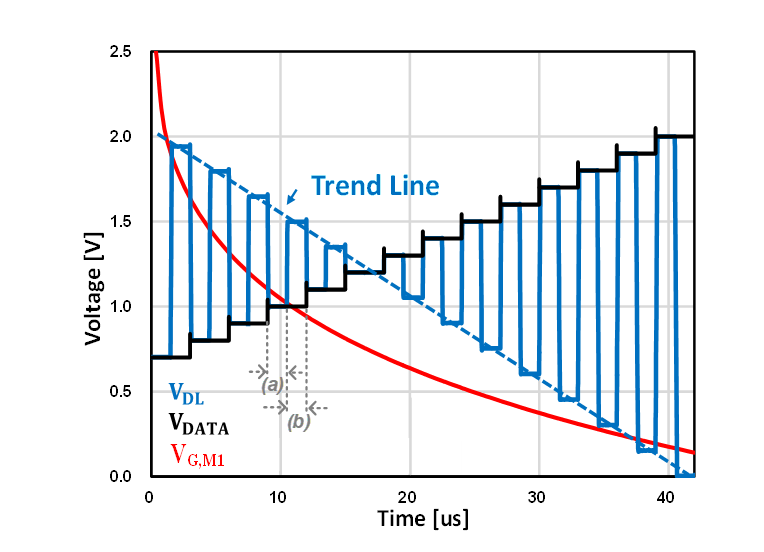(4)
$V_{D L}=-\frac{C_{1}}{C_{2}} \cdot\left(V_{R E F}-V_{D A T A}\right)+V_{D A T A}$

From (4), V$_{\mathrm{DL}}$ can be linearly initialized and the polarity between V$_{\mathrm{DL}}$ and V$_{\mathrm{DATA}}$ is different because of the p-type TFT (M$_{1}$).

Fig. 5 shows the simulation result when increasing V$_{\mathrm{DATA}}$ as a sample-and-hold signal. With an analog buffer, V$_{\mathrm{DL}}$ is ideally the same as V$_{\mathrm{DATA}}$ in the first initialization phase, and the OPA amplifies the sampled signal (V$_{\mathrm{REF}}$-V$_{\mathrm{DATA}}$) as much as the capacitance ratio (C$_{1}$/C$_{2}$) in the second initialization phase. Although the trend line of V$_{\mathrm{DL}}$ was not precisely identical to the gate voltage of M$_{1 }$(V$_{\mathrm{G,M1}}$), the pull-up time was significantly reduced because V$_{\mathrm{DL}}$ was initialized as the voltage, which was close to the V$_{\mathrm{G,M1}}$ flowing the programmed current in the compensation time. In addition, controlling C$_{1}$/C$_{2}$ and V$_{\mathrm{REF}}$ enabled us to optimize the initialized V$_{\mathrm{DL}}$s in the range of programmed currents.

The OPA structure in the proposed circuit was designed as a two-stage operational trans-conductance amplifier (OTA) and enhanced slew-rate source follower (4), as shown in Fig. 6. The primary design consideration for stable operation in different phases was F$_{\mathrm{P.OPA}}$. In the initialization phases, F$_{\mathrm{P.OPA}}$ was located in the output of the input stage and appeared in a low-frequency region by adopting the pole-control block. In the compensation phase,F$_{\mathrm{P.OPA}}$ appeared in a high-frequency region for a sizable phase margin in the compensation feedback loop by eliminating the pole-control block. Therefore, F$_{\mathrm{P.OPA}}$ can be controlled by the pole-control block, which is identical to the compensation block in an OTA. The OPA was designed by using 110-nm CMOS, and the total static current was 10 ${\mathrm{\mu}}$A/channel.

IV. Simulation Results

Table 1. Performance summary of the low-luminance compensation current driver

 Parameters (5) (6) This work Resolution 1920×1080 3840×2160 3840×1200 Panel Loads (RP/CP) 4 kΩ / 90 pF 30 kΩ / 30 pF 1.5 kΩ / 25 pF Process 350 nm CMOS 180 nm CMOS 110 nm CMOS One-row time 6 μs 7.7 μs 10 μs Driving Current 10 nA (meas.) 39 nA (meas.) 2 nA (sim.) Current consumption /channel 4.5 μA - 10 μA

Fig. 7 shows the transient simulation results with TFT SPICE models in one-row time. The one-row time included the first initialization phase (1.5 ${\mathrm{\mu}}$s), the second initialization phase (1.5 ${\mathrm{\mu}}$s), and the compensation phase (7 ${\mathrm{\mu}}$s). Thanks to the initialization phases for the data and feedback lines, additional pull-up times in the compensation phase were eliminated, and the proposed structure had the driving ability to compensate for TFT variations at the lowest programmed current of 2 nA within 10 ${\mathrm{\mu}}$s of one-row time. Although the PM was degraded as the programmed current increased because of constant F$_{\mathrm{P.OPA}}$, the proposed structure compensated up to 50 nA of current with a 1% error rate. Table 1 compares the performance of the proposed circuit with other state-of-the-art works (5,6). It should be noted that the performances of other works are based on the measurement results, and those of the proposed circuit is based on the simulation results. Within 10 ${μ}$s of one-row time, the lowest 1LSB driving current (=2 nA) settled down. Therefore, high speed and small-current drivability can be achieved by the proposed initialization technique and feedback characteristics of FB-Gm.

V. CONCLUSIONS

In this paper, a low-luminance compensation current driver that uses an initialization technique with FB-Gm was proposed for AMOLED displays to drive currents accurately. Conventional FB-Gm structures have driving-speed limitations because of parasitic components, creating crucial slew time. Therefore, with the proposed FB-Gm with initialization technique consisting of only two additional capacitors and eight switches, we achieved that both a good low-current drivability from the merit of FB-Gm and a high driving speed with the charge transfer circuit. The experimental results with TFT SPICE models showed that the proposed current driver can compensate the lowest programmed current of 2 nA within 10 ${\mathrm{\mu}}$s of one-row time.

ACKNOWLEDGMENTS

This work was supported in part by the LG Display Co. Ltd and in part by the MSIT(Ministry of Science and ICT), Korea, under the ITRC(Information Technology Research Center) support program(IITP-2021-2018-0-01421) supervised by the IITP(Institute for Information & Communications Technology Planning & Evaluation).

REFERENCES

1
Tai Y., Huang S., Chen W., Chao Y., Chou Y., Peng G., Dec 2007, A Statistical Model for Simulating the Effect of LTPS TFT Device Variation for SOP Applications, in Journal of Display Technology, Vol. 3, No. 4, pp. 426-4332
Ono S., Miwa K., Maekawa Y., Tsujimura T., March 2007, $V_{T}$ Compensation Circuit for AM OLED Displays Composed of Two TFTs and One Capacitor, in IEEE Transactions on Electron Devices, Vol. 54, No. 3, pp. 462-4673
Amiri M., Zamir A. R., Shahin S., Ashtiani S. J., 2012, A novel 3-TFT AMOLED pixel driver for threshold voltage shift compensation, 2012 IEEE International Conference on Electronics Design Systems and Applications (ICEDSA) Kuala Lumpur, pp. 121-1244
Kenney J. G., Rangan G., Ramamurthy K., Temes G., Feb 1995, An enhanced slew rate source follower, in IEEE Journal of Solid-State Circuits, Vol. 30, No. 2, pp. 144-1465
Jeon J., 2008, A Direct-Type Fast Feedback Current Driver for Medium-to Large-Size AMOLED Displays, 2008 IEEE International Solid-State Circuits Conference - Digest of Technical Papers San Francisco CA, pp. 174-6046
Bang J., April 2016, A Hybrid AMOLED Driver IC for Real-Time TFT Nonuniformity Compensation, in IEEE Journal of Solid-State Circuits, Vol. 51, No. 4, pp. 966-978Author

received B.S. in semiconductor science from Dong-guk University, Seoul, South Korea, 2019.

He is currently pursuing the M.S. degree in semiconductor science with Dongguk University, Seoul, Republic of Korea.

His current research interests include low power CMOS image sensor and OLED compensation circuit design.

received B.S. and M.S. degrees in semiconductor science from Dongguk University, Seoul, Republic of Korea, 2016 and 2018, respectively.

He is currently pursuing the Ph.D. degree in semiconductor science with Dongguk University, Seoul, Republic of Korea.

His current research interests include thermal-aware FinFET circuit design and OLED compensation circuit design.

received his B.S and M.S degrees from the Department of Information Display at Kyunghee University, Republic of Korea, in 2015 and 2017.

He is currently an engineer with Panel Driving Team, LG Display, Seoul, Republic of Korea from 2017.

His current research interests include driving methods for LCD and OLED Display.

received his B.S degrees from the Department of Electrical Engineering at Kwang-woon University, Republic of Korea, in 2011.

He is currently an engineer with Panel Driving, LG Display, Seoul, Republic of Korea from 2011.

His current research interests include driving methods for LCD and OLED Display.

received B.S. and M.S. degrees in semiconductor science from Dongguk University, Seoul, Republic of Korea, 2001 and 2003, respectively and the Ph.D. degree in electrical and computer engineering from the School of Electrical and Computer Engineering, Purdue University, West Lafayette, IN, USA, in 2013.

From 2003 to 2008, she was an engineer with Image Development Team of System LSI division, Samsung electronics, Yongin, Republic of Korea.

From 2013 to 2017, she was a staff engineer with Qualcomm Corporate Research and Development, San Diego, CA. USA.

She is currently an assistant professor with the semiconductor science, Dongguk University, Seoul Republic of Korea.

Her current research interests cover low-power CMOS image sensor, OLED compensation circuit design, and thermal-aware FinFET circuit design.